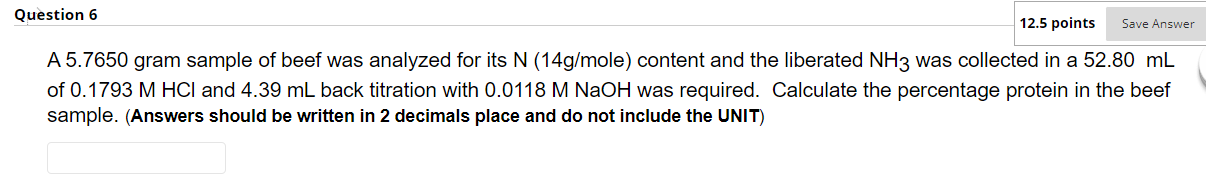Home / Expert Answers / Chemistry / a-5-7650-gram-sample-of-beef-was-analyzed-for-its-mathrm-n-14-mathrm-g-mathrm-mol-pa971

# (Solved): A $$5.7650$$ gram sample of beef was analyzed for its $$\mathrm{N}(14 \mathrm{~g} / \mathrm{mol ...A \( 5.7650$$ gram sample of beef was analyzed for its $$\mathrm{N}(14 \mathrm{~g} / \mathrm{mole})$$ content and the liberated $$\mathrm{NH}_{3}$$ was collected in a $$52.80 \mathrm{~mL}$$ of $$0.1793 \mathrm{M} \mathrm{HCl}$$ and $$4.39 \mathrm{~mL}$$ back titration with $$0.0118 \mathrm{M} \mathrm{NaOH}$$ was required. Calculate the percentage protein in the beef sample. (Answers should be written in 2 decimals place and do not include the UNIT)

We have an Answer from Expert